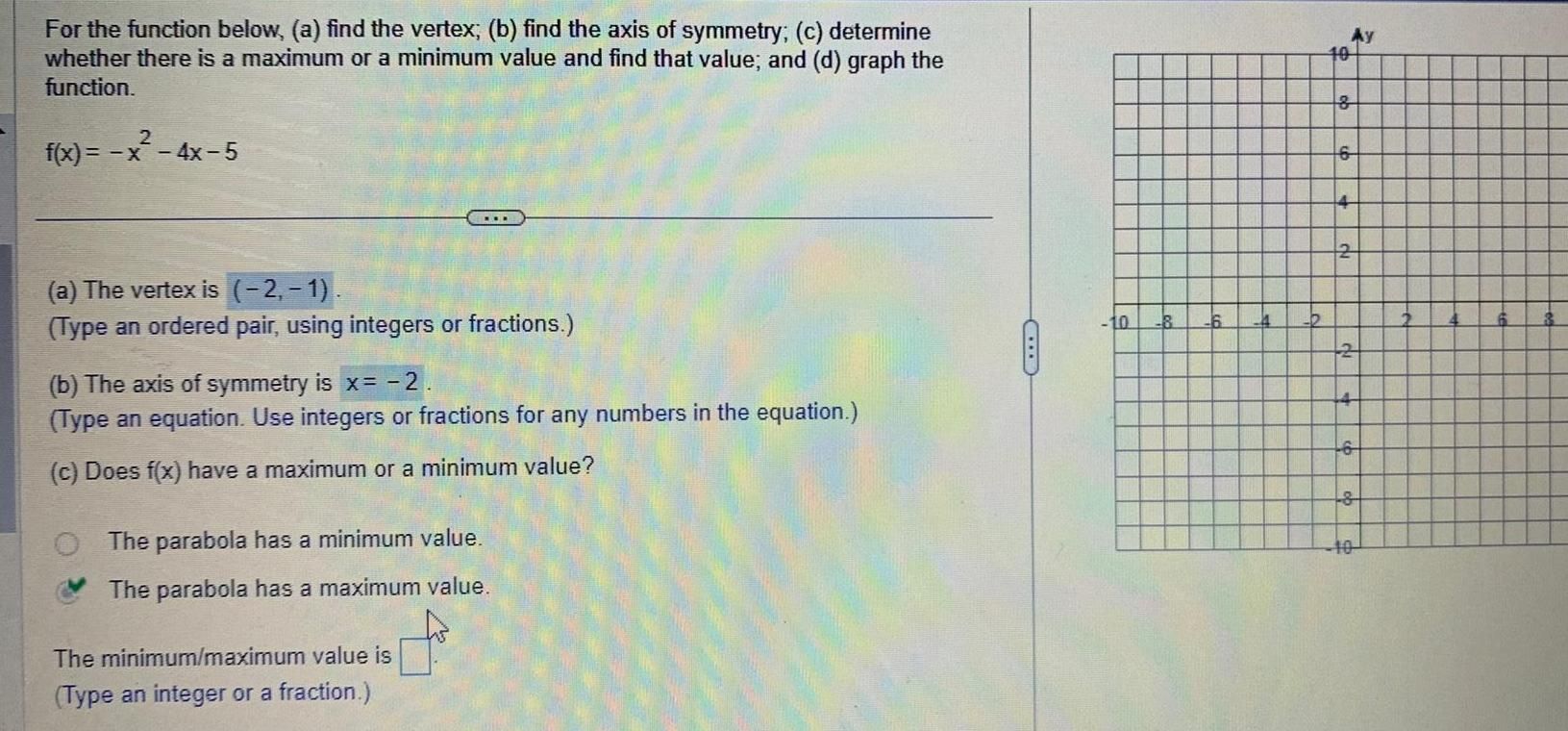Question:

# For the function below a find the vertex b find the axis of

Last updated: 9/19/2023For the function below a find the vertex b find the axis of symmetry c determine whether there is a maximum or a minimum value and find that value and d graph the function 2 f x x 4x 5 a The vertex is 2 1 Type an ordered pair using integers or fractions b The axis of symmetry is x 2 Type an equation Use integers or fractions for any numbers in the equation c Does f x have a maximum or a minimum value The parabola has a minimum value The parabola has a maximum value The minimum maximum value is Type an integer or a fraction 10 8 6 4 Ay 10 8 6 4 2 2 4 6 8